## General Question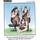# Can you teach me what the function of the variable E, in university math is with using logic and not complex math?

Asked by talljasperman (21863) October 27th, 2013

All I know is that E is equal to 2.5 , but I don’t know the back ground to the use for it. Also please include the philosophy or history of e and what can it be used for out of school.
still confused

Observing members: 0Composing members: 0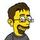Well, from your last question, it looked like you typed it in wrong to begin with. Try this

But asking to explain complex math without using complex math is beyond me. Maybe this will help.

As for it’s uses outside of school, try calculating compound interest and you will run into e

jerv (31044)“Great Answer” (6) Flag as…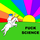When Wikipedia is too complex, sometimes Simple English Wikipedia can help.

When it comes to understanding exactly what it is and why it is important, this should mostly come from experience, in my opinion. Hence, working with it often and coming across it – this happens when you work with e.g. logarithms a lot. Admittedly, not everybody will be doing so and hence chances are that you will not use if outside of school, but there are more fields than you would imagine at first that do use it.

As for where the number comes from, maybe this page helps as well.

Edit: @jerv‘s article is excellent, a great guide to understand what e actually means.

Vincentt (8079)“Great Answer” (5) Flag as…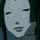ARE_you_kidding_me (19773)“Great Answer” (3) Flag as…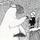It’s not a variable. It’s a constant.

glacial (12140)“Great Answer” (3) Flag as…e is related to exponential growth. For example, if a bank gives interest at 3 percent compounded yearly then if you deposit principal p, the amount of money in the bank after t years is p(1.03)^t. If the money is compounded n times a year then the amount of interest per time period is ,03/n and the number of compounding periods is nt, so the amount of money at the end of t years is (1 + .03/n)^nt.

Now fantasize that you get 100% interest and that the compounding is done instantaneously. For a deposit of \$1, the amount of money in the bank at the end of 1 year is the limit as n goes to infinity of (1+1/n)^n. That is the number e. It turns out that there are a number of formulas related to calculus that work out very nicely using e as the base for exponentiation.

LostInParadise (28140)“Great Answer” (1) Flag as…or